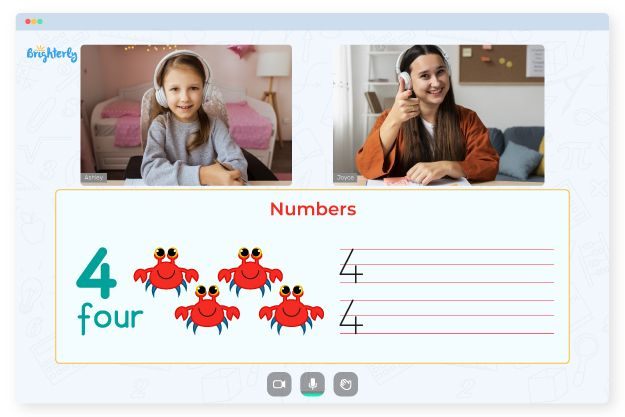# Graphing Inequalities Worksheet

The graphing inequalities worksheet helps students learn how to graph inequalities with simple steps. You’ll also have access to graphing inequalities worksheet algebra 2 and graphing inequalities worksheet answer keys to solve practice questions.

## How to Graph an Inequality

Here’s how to graph an Inequality:

• Plot the line for the inequality on a set of axes.
• Determine the boundary points where the line meets the boundary of the graph.
• Shade the region that meets the conditions of inequality.
• Label the solution set, which is the region that satisfies the inequality.

The shading direction (above or below the line) depends on the inequality symbol used. For example, if the inequality is “y > x + 2,” shade the region above the line, and if the inequality is “y < x + 2,” shade the region below the line.

The line representing the inequality can be linear or nonlinear, depending on the equation. To graph a linear inequality, you can use the slope-intercept form (y = mx + b) or the point-slope form (y – y1 = m(x – x1)) to find the line equation and graph it on a set of axes. For nonlinear inequalities, you can use a graphing calculator to find and graph the solution set.

Math for Kids

Is Your Child Struggling With Math?
1:1 Online Math Tutoring## Graphing Inequalities with Two Variables

To graph an inequality with two variables, you need to follow these steps:

• Plot the line for the inequality on a set of axes, with one variable on the x-axis and the other variable on the y-axis.
• Determine the boundary points where the line meets the boundary of the graph.
• Shade the region that meets the conditions of inequality.
• Label the solution set, which is the region that satisfies the inequality.

## Graphing Absolute Value Inequality

Follow the steps below to graph absolute value inequality:

• Isolate the absolute value expression on one side of the inequality.
• Split the inequality into two separate cases, one where the expression inside the absolute value is positive and one where it is negative.
• Plot the lines corresponding to each case and shade the appropriate region.
• Label the solution set, which is the region that satisfies the inequality.### Graphing Inequalities Worksheet PDF

Graph Inequalities Worksheet### Graphing Inequalities Worksheet PDF

Graphing Absolute Value Inequalities Worksheet### Graphing Inequalities Worksheet PDF### Graphing Inequalities Worksheet PDF

Graphing Inequalities Worksheet

Need help with Numbers?• Is your child having difficulties with understanding numbers?
• An online tutor could provide the necessary support.

Does your child struggle to grasp the concept of numbers? Try lessons with an online tutor.